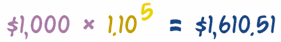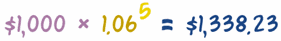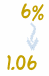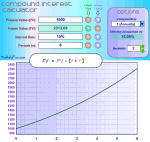# 复利0 （现在）
￥1,000.00
（￥1,000.00 × 10% = ） ￥100.00
￥1,100.00
1
￥1,100.00
（￥1,100.00 × 10% = ）) ￥110.00
￥1,210.00
2
￥1,210.00
（￥1,210.00 × 10% = ） ￥121.00
￥1,331.00
3
￥1,331.00
（￥1,331.00 × 10% = ） ￥133.10
￥1,464.10
4
￥1,464.10
（￥\$1,464.10 × 10% = ） ￥146.41
￥1,610.51
5
￥1,610.51

1. 计算利息（= "初始借贷额" × 利率）
2. 把利息加到 "初始借贷额" 上来得到年底的 "终极借贷额"
3. 年底的 "终极借贷额" 是下一年的 "初始借贷额"

## 做个公式

41,000.00 + （￥1,000.00 × 10%）= ￥1,100.00所以这个： ￥1,000 +（￥\$1,000 x 10%）= ￥1,000 + ￥100 = ￥1,100 和这个是相同的： ￥1,000 × 1.10 = ￥1,100

10% = 10/100 = 0.10

• 10% ⇒ 1.0 ⇒ 0.10
• 12% ⇒ 1.2 ⇒ 0.12
• 6% ⇒ 0.6 ⇒ 0.06

1. 把 "初始借贷额" 乘以 （1 + 利率）来得到 "终极借贷额"

……公式在任何年份都适用！

• 我们可以这样求下一年的利息：￥1,100 × 1.10 = ￥1,210
• 再下一年：￥1,210 × 1.10 = ￥1,331
• 等等……￥1,000 × 1.10 × 1.10 × 1.10 × 1.10 × 1.10 = ￥1,610.51## 公式（看到和上面的计算是一样的吗？PV = ￥1,000、 r = 0.10, n = 5、 FV = ￥1,610.51）

FV = PV × (1+r)n

PV = 现值
r = 年利率
n = 期数这就是复利的基本公式。 它非常重要，值得牢记。

### 例子

…… 如果年期是 15年呢？……改变 "n" 的值：……如果年期是 5年，而利率是 6% 又怎么样？我们把 6% 代入公式::……20年，8%？你自己来！

## "反过来"求现值PV = FV / (1+r)n

PV = ￥2,000 / (1+0.10)5 = ￥2,000 / 1.61051 = ￥1,241.84

PV = ￥10,000 / (1+0.08)10 = ￥10,000 / 2.1589 = ￥4,631.93

## 复利计算期

FV = PV × (1+r)n = ￥1,000 × (1.01)12 = ￥1,000 × 1.12683 = ￥1,126.83 = 需要还的款项

FV = PV × (1+r/n)n = ￥1,000 × (1 + 6%/12)12 = ￥1,000 × (1.005)12 = ￥1,000 × 1.06168…… = ￥1,061.68 = 需要还的款项

## APR （年度百分率）房贷广告通常都相当复杂（有时是故意复杂的！），时常你会看到 "APR" （年度百分率）。 APR 就是 "年度百分率"…… 它的意思是你一年里实际上要支付多少 （包括复利、其他收费等等）。 其实是 6.335%

## 歇会儿！

• 利率，如果已知现值、终值和期数。
• 期数，如果已知现值、终值和利率

## 求利率

r =（FV / PV）1/n − 1注意："1/n" 是个 分数指数，先计算 1/n，然后把结果作为指数输入计算器。 例如，20.2 是这样输入的：2，"x^y"， 0，.， 2，=

r =（￥2,000 / ￥1,000）)1/5 − 1 =（2）0.2 − 1 = 1.1487 − 1 = 0.1487

0.1487 就是 14.87%

r =（￥5,000 / ￥1,000）1/20 − 1 =（5）0.05 − 1 = 1.0838 − 1 = 0.0838

0.0838 就是 8.38%

## 求期数

n = ln(FV / PV) / ln(1 + r)好计算器都会有 "ln" 函数功能。 你也可以用 log，但不能两个一起用。

n = ln( \$2,000 / \$1,000 ) / ln( 1 + 0.10 ) = ln(2)/ln(1.10) = 0.69315/0.09531 = 7.27

n = ln( \$10,000 / \$1,000 ) / ln( 1 + 0.05 ) = ln(10)/ln(1.05) = 2.3026/0.04879 = 47.19

47年！这不稀奇，因为金额大了十倍，而利率只有 5%。## 总结

 FV = PV (1+r)n 求终值，其中： FV = 终值、 PV = 现值、 r = 利率（以小数表达）、 n = 期数 重排这个公式（见导出复利公式），我们便可以从已知任何三个去求第四个变量： PV = FV(1+r)n 求现值，已知终值、利率和期数。 r = (FV/PV)(1/n) − 1 求利率，已知现值、终值和期数。 n = ln(FV / PV)ln(1 + r) 求期数，已知现值、终值和利率（注意：ln 是 自然对数函数）